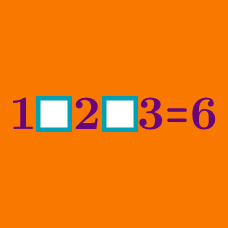Logic

Operator Search: Level 2 Challenges

$\large 1 \, \square \, 2 \, \square \, 3 \, \square \, 4 \, \square \, 5 \, \square \, 6 \, \square \, 7 \, \square \, 8 = 9$

There are $2^7 =128$ ways in which we can fill the squares with $+, -$.

How many ways would make the equation true?

Note: You are not allowed to use parenthesis.

$\large 3 \; \square \; 3 \; \square \; 3 \; \square \; 3$

Fill in the boxes above with any of the four mathematical operators ( $+, -, \times , \div$ ). Which of the following cannot be a resultant number?

Note: Order of operations (BODMAS) applied.

$\large 1 \; \square \; 2 \; \square \; 3\; \square \; 4\; \square \; 5 \; \square \; 6 \; \square \; 7 \; \square \; 8 \; \square \; 9 = 33$

Seven of the eight "$\square$"s above are filled with "$+$", and the other one with "$-$".

Before which integer should the "$-$" sign be placed to make the equation true?

$\large 18 \ \square \ 12 \ \square \ 4 \ \square \ 5 = 59$

Replace each $\square$ with one of $+,-,\times,\div$ to make the equation true.

• $1$ represents $+$
• $2$ represents $-$
• $3$ represents $\times$
• $4$ represents $\div$

Example : If your answer is $+, -, \times$ then enter the answer as $123$.

$\Huge 1\ \ \ \ 4 \ \ \ \ 9 \ \ = \ \ 16$

Is it possible to make this equation true by inserting the appropriate operations? Any operations and functions can be used.

×